# Permutation and Combinations (Part - 3) Notes | Study Business Mathematics and Logical Reasoning & Statistics - CA Foundation

## CA Foundation: Permutation and Combinations (Part - 3) Notes | Study Business Mathematics and Logical Reasoning & Statistics - CA Foundation

The document Permutation and Combinations (Part - 3) Notes | Study Business Mathematics and Logical Reasoning & Statistics - CA Foundation is a part of the CA Foundation Course Business Mathematics and Logical Reasoning & Statistics.
All you need of CA Foundation at this link: CA Foundation

SUMMARY

⇒ Fundamental principles of counting

(a) Multiplication Rule: If certain thing may be done in ‘m’ different ways and when

it has been done, a second thing can be done in ‘n ‘ different ways then total number

of ways of doing both things simultaneously = m × n.

(b) Addition Rule: It there are two alternative jobs which can be done in ‘m’ ways

and in ‘n’ ways respectively then either of two jobs can be done in (m + n) ways.

⇒ Factorial: The factorial n, written as n! or n , represents the product of all integers from 1 to n both inclusive. To make the notation meaningful, when n = o, we define o! or o

= 1.

Thus, n! = n (n – 1) (n – 2) ….. …3.2.1

Permutations: The ways of arranging or selecting smaller or equal number of persons

or objects from a group of persons or collection of objects with due regard being paid to

the order of arrangement or selection, are called permutations.

The number of permutations of n things chosen r at a time is given by

nPr =n ( n – 1 ) ( n – 2 ) … ( n – r + 1 )

where the product has exactly r factors.

Circular Permutations: (a) n ordinary permutations equal one circular permutation.

Hence there are nPn/ n ways in which all the n things can be arranged in a circle. This

equals (n–1)!.

(b) the number of necklaces formed with n beads of different colours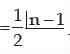1. Number of permutations of n distinct objects taken r at a time when a particular object is not taken in any arrangement is n–1pr.
2. Number of permutations of r objects out of n distinct objects when a particular object is always included in any arrangement is r. n–1pr-1.

⇒ Combinations: The number of ways in which smaller or equal number of things are

arranged or selected from a collection of things where the order of selection or

arrangement is not important, are called combinations.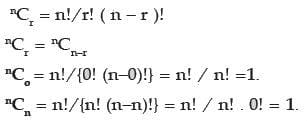(a) nCr has a meaning only when r and n are integers 0≤ r ≤ n and nCn–r has a meaning only when 0 ≤ n – r ≤ n.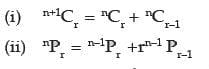Permutations when some of the things are alike, taken all at a time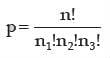Permutations when each thing may be repeated once, twice,…upto r times in any

arrangement = n!.

⇒ The total number of ways in which it is possible to form groups by taking some or all of

n things (2n –1).

The total, number of ways in which it is possible to make groups by taking some or all

out of n (=n1 + n2 + n3 +…) things, where n1 things are alike of one kind and so on, is

given by { (n1 + 1) ( n2 + 1) ( n3 + 1)…} –1
⇒ The combinations of selecting r1 things from a set having n1 objects and r2 things from a set having n2 objects where combination of r1 things, r2 things are independent is given

by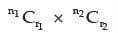The document Permutation and Combinations (Part - 3) Notes | Study Business Mathematics and Logical Reasoning & Statistics - CA Foundation is a part of the CA Foundation Course Business Mathematics and Logical Reasoning & Statistics.
All you need of CA Foundation at this link: CA FoundationUse Code STAYHOME200 and get INR 200 additional OFF

### Up next## Business Mathematics and Logical Reasoning & Statistics

81 videos|101 docs|87 tests

### Up nextTrack your progress, build streaks, highlight & save important lessons and more!

,

,

,

,

,

,

,

,

,

,

,

,

,

,

,

,

,

,

,

,

,

;